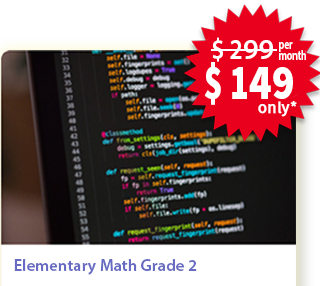## High School Algebra## Private Lessons

• 2 sessions every week
• Each session is of 1 hour

#### Overview

Aligned with the common core curriculum of high school Algebra, this course introduces the concepts of expressions, algebraic manipulations, equations & inequalities and connections to functions and modelling.

#### Curriculum

###### Seeing Structure in Expressions

• Interpret the structure of expressions
• Write expressions in equivalent forms to solve problems

###### Arithmetic with Polynomials and Rational Functions

• Perform arithmetic operations on polynomials
• Understand the relationship between zeros and factors of polynomials
• Use polynomial identities to solve problems
• Rewrite rational functions

###### Creating Equations

• Create equations that describe numbers or relationships

###### Reasoning with Equations and Inequalities

• Understand solving equations as a process of reasoning and explain the reasoning
• Solve equations and inequalities in one variable
• Solve systems of equations
• Represent and solve equations and inequalities graphically

### Enroll for Math Private LessonsEnroll today to book your slot!

*Weekly 2 classes. Only limited seats available, Enroll Today.